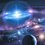# ${ x }^{ 3 }+{ y }^{ 3 }={ z }^{ 3 }$

According to Fermat's Last Theorem, ${ a }^{ n }+{ b }^{ n }={ c }^{ n }$ have no solutions in positive integers, if n is an integer greater than 2.

But I can (hopefully) only prove ${ x }^{ 3 }+{ y }^{ 3 }={ z }^{ 3 }$.

Expand ${ x }^{ 3 }+{ y }^{ 3 }={ z }^{ 3 }$ and you will get $(x+y)({ x }^{ 2 }-xy+{ y }^{ 2 })$

So, ${ x }^{ 3 }+{ y }^{ 3 }$= $(x+y)({ x }^{ 2 }-xy+{ y }^{ 2 })$

Any integer can be expressed as $x+y$.

Hence, let $z$ be $x+y$

$(x+y)({ x }^{ 2 }-xy+{ y }^{ 2 })$=${ (x+y) }^{ 3 }$

Dividing both sides by $(x+y)$

${ x }^{ 2 }-xy+{ y }^{ 2 }$=${ (x+y) }^{ 2 }$

Expanding ${ (x+y) }^{ 2 }$, you will get ${ x }^{ 2 }+2xy+{ y }^{ 2 }$

Simplifying the equation, $-xy=2xy$

$0=3xy$

Hence, $x=0$ and $y=$any integer.

OR

$x=$any integer and $y=0$.

But anybody know that $0$ can be a solution.

Note that $0$ is a neutral number

Now consider the case, $z\neq x+y$

Assume $z=k(x+y)$

Where $k$ is any integer.

Using graph theory it is easy to realise that there are no rational solutions.

If interested, view Leonhard Euler's proof of this.Note by Luke Zhang
6 years, 6 months ago

This discussion board is a place to discuss our Daily Challenges and the math and science related to those challenges. Explanations are more than just a solution — they should explain the steps and thinking strategies that you used to obtain the solution. Comments should further the discussion of math and science.

When posting on Brilliant:

• Use the emojis to react to an explanation, whether you're congratulating a job well done , or just really confused .
• Ask specific questions about the challenge or the steps in somebody's explanation. Well-posed questions can add a lot to the discussion, but posting "I don't understand!" doesn't help anyone.
• Try to contribute something new to the discussion, whether it is an extension, generalization or other idea related to the challenge.

MarkdownAppears as
*italics* or _italics_ italics
**bold** or __bold__ bold
- bulleted- list
• bulleted
• list
1. numbered2. list
1. numbered
2. list
Note: you must add a full line of space before and after lists for them to show up correctly
paragraph 1paragraph 2

paragraph 1

paragraph 2

[example link](https://brilliant.org)example link
> This is a quote
This is a quote
    # I indented these lines
# 4 spaces, and now they show
# up as a code block.

print "hello world"
# I indented these lines
# 4 spaces, and now they show
# up as a code block.

print "hello world"
MathAppears as
Remember to wrap math in $$ ... $$ or $ ... $ to ensure proper formatting.
2 \times 3 $2 \times 3$
2^{34} $2^{34}$
a_{i-1} $a_{i-1}$
\frac{2}{3} $\frac{2}{3}$
\sqrt{2} $\sqrt{2}$
\sum_{i=1}^3 $\sum_{i=1}^3$
\sin \theta $\sin \theta$
\boxed{123} $\boxed{123}$

Sort by:

@Calvin Lin I think you would like this.

Seems legid but it means that $-1=2$. I can't find the error and how to resolve it.

- 6 years, 6 months ago

Only hitch in the solution is that $3xy = 0$ does not imply that BOTH $x = 0$ and $y = 0$. Only one the statements has to be true.

@Julian Poon . I don't see the problem you're having. Where does $-1 = 2$? Or has the note been edited?

- 6 years, 5 months ago

I dont really think theres a problem with this but @Luke Zhang says that this step isnt valid even though this step is valid to me:

$-xy=2xy$

However, this step (to me) isn't valid:

$-1=2$

Since this proof does not have the division by $0$, I don't think there is a problem.

- 6 years, 5 months ago

For that part you can't prove -1=2 as you will be dividing both sides by 0 which is invalid. No problem with that prove. Just lacking 3 more cases.

- 6 years, 5 months ago

- 6 years, 6 months ago

The Boh is right.

- 6 years, 6 months ago

The BOH?

- 6 years, 5 months ago

I still dont think that there is any error in this cause there is no division by $0$. It is just proving $x$ and/or $y$ is $0$.

- 6 years, 5 months ago

Yes Julian But I said both x and y bust be 0

- 6 years, 5 months ago

THats why there isnt an error.

- 6 years, 5 months ago

But there is! As I claimed both x and y must be 0 Why not ask Calvin to vet it?

- 6 years, 5 months ago